Russian Journal of Earth Sciences
Vol. 5, No. 5, October 2003

# Wavelet analysis of secular geomagnetic variations

N. M. Rotanova, T. N. Bondar, and E. V. Kovalevskaya

Institute of Terrestrial Magnetism, Ionosphere and Radio Wave Propagation, Russian Academy of Sciences, Troitsk

### Abstract

Temporal characteristics of secular variations ( SV ) over the last hundred of years are studied using the wavelet transformation, providing estimates of dispersion and energy parameters of the process analyzed. The presence of abrupt geomagnetic field variations of the intraterrestrial origin in periods of 1969-1970 and 1989-1990 is confirmed. The SV process is, on the whole, nonfractal, and only two largest scales can be regarded as monofractal. The SV scale diagrams obtained from the wavelet analysis not only revealed singularities in the SV field but also provided constraints on variations in its energy parameters throughout the time interval studied. On the basis of the SV phase portrayal constructed in our study, the limits of the SV dynamic system are estimated to be 2

### 1. Introduction

The magnetic field and its secular variations ( SV ) observed on the Earth's surface are a complex function of space and time. The identification of regular patterns in the geomagnetic field relies not only on the acquisition of new experimental data but also on the application of more effective methods of analysis.

As was shown in [Astafyeva, 1996; Dremin et al., 2001; Dyakonov, 2002], the Fourier representation of a process and the application of the maximum entropy method [Cay and Marple, 1981] are ineffective for the analysis of processes with local features. This is due to the fact that the basis function inherent in these methods, being defined in the entire space, is a smooth and strictly periodic function. Such a function can provide constraints only on characteristic periods and intensity of their variations but not on the temporal localization and characteristic scales. The wavelet analysis [Daubechies, 1992; Mallat, 1989; Meyer, 1992] is free from these disadvantages. The wavelet transformation is based on the use of an essentially new basis (a wavelet) represented by a short wave with a zero mean and localized on the temporal (spatial) axis. Wavelets are constructed with the use of special basis functions enabling the representation of a 1-D process in the time-frequency (scale) plane and the analysis of its spectral properties as a function of time or scale.

Abroad, the use of wavelets dates back to the early 1990s. However, recently the interest in wavelets has sharply increased in Russia as well. Presently, a number of reviews have been published in Russian journals [Astafyeva, 1996; Dremin et al., 2001] and monographs devoted to wavelets have been translated [Chui, 2001; Daubechies, 2001]. Wavelets have also been widely used in geophysics [Alexandrescu et al., 1995; Alperovich and Zheludev, 1998; Gilbert et al., 1998; Ippolitov et al., 2001; Ivanov and Rotanova, 2000; Rotanova et al., 2002]. The goal of our work is to apply the wavelet analysis to the study of the temporal structure of the geomagnetic secular variations.

### 2. Method of Wavelet Analysis of Temporal Series

The wavelet transformation aims at the identification of the fine structure of a temporal or spatial series. In the general case, the family of analyzing wavelets is constructed in the form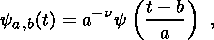(1)

where a is the scale parameter, b is the shift parameter and n is the normalizing coefficient. Then, the continuous wavelet transformation of a discrete series fn(t) is defined as the convolution of this series with the wavelet y(a, b):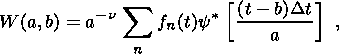(2)

where y (a, b) is the basis wavelet function and the asterisk means a complex conjugation.

In our numerical calculations, we utilized the MHAT-wavelet [Rotanova et al., 2002], which has a narrow energy spectrum and two equal zero moments. The analytical expression for such a moment is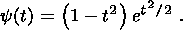(3)

The wavelet transformation of the temporal series fn(t) yields a 2-D set of the coefficients W (a, b) providing constraints on the contributions of components of various scales and their variations with time. Based on the known values of W (a, b), it is easy to estimate some energy characteristics. Among the latter, the simplest and useful parameter is the energy density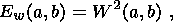(4)

which characterizes the energy levels of the study process f (t) in the space (a, b).

Since an analogue of the Parseval equality exists for the wavelet transformation, the total energy of the process f (t) can be written, for real functions, through the amplitudes of this transformation as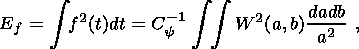(5)

where C-1y is the normalizing coefficient similar to the coefficient in the Fourier transformation. The confidence interval of the energy spectrum of the wavelet transformation is determined as the probability that, at given parameters a and b, the true spectrum lies within the interval of the estimated wavelet power spectrum,(6)

where |W (a, b)|2 is the true wavelet spectrum, n = N-1, N is the number of observation points, p is the significance level, and x2n (p/2) is the chi-square distribution with n degrees of freedom.

A series of estimates averaged along the shear parameter b or along the scale coefficient a. In the first case, we have the time-averaged power spectrum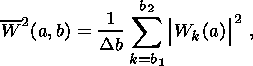(7)

where Db = b2-b1+1 is the number of averaging points on the horizontal axis b. In the second case, the summation is performed over the scale axis a, and the scale-averaged spectrum has the form(8)

Since we examine the series varying with time, the wavelet coefficients are also time dependent. A natural measure for fluctuations of the coefficients is the variance distributions over various scales. The variance at each scale a is calculated by the formula(9)

where N is the number of wavelet coefficients on a scale a in a given time interval.

The wavelet analysis is most suitable for studying the fractal behavior of a time series. This is due to the fact that the wavelet transformation provides a signal on various scales obtained by calculating the scalar product of the analyzing wavelet by the signal analyzed. As regards the wavelet coefficients, this implies the power-law behavior of their higher-order moments as a function of scale. The wavelet transformation allows one to calculated the higher-order moments Zq on various scales a:(10)

where maximum values of |W (a, b)| are summed. Dremin et al. [ showed that, in the case of a fractal process, this sum should vary as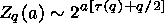(11)

or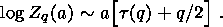(12)

As follows from (12), the necessary condition for the self-similarity of the process is a linear dependence of log Zq on the scale a. If this condition is met, the dependence of the function t on the rank of the moment q defines the given process as mono- or multifractal. Monofractal processes have a single dimension, whereas multifractal processes are characterized by a set of such dimensions.

Finally, we dwell on a method for constructing the phase space portrayal and the attractor structure for the process studied. For this purpose, we make use of a procedure known in literature as the Takens procedure (in the theory of embedding of nonlinear dynamic systems [Takens, 1981]). This procedure reduces to the following.

Component vectors of the system state are constructed from the original temporal series SV(t):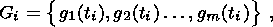(13)

where gk (ti) = g[ti + (k + 1)l] and l is the retarding time. The spatial distribution of the vectors gm(ti) forms the reconstructed phase space of the dimension m.

### 3. Results Obtained from the Wavelet Analysis of the SV Temporal Series of the Geomagnetic Field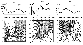Figure 1
The wavelet analysis was applied to SV temporal series of the geomagnetic field recorded in observatories. Results of such a transformation of the three components SVx, SVy and SVz measured in the Nimegk magnetic observatory are shown in Figure 1. The shift parameter and the time of the series analyzed are plotted on the horizontal axis in this figure and the scale coefficient a, increasing in the upward direction, is plotted on its vertical axis. Figure 1a presents the field SVx and its wavelet transform. Four large-scale features on scales of ~10 to ~20 years are seen in its upper part. Quite a different pattern observed in its lower part is represented by separate small-scale structures with small amplitudes and time scales of less than 10 years. The wavelet transform of the vertical component SVz shown in Figure 1b exhibits two large features differing in scale and in amplitude. The scale of the positive feature is ~40 years, and that of the negative one is about 20 years. A few local structures on scales of less than 10 years are also recognizable.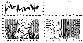Figure 2
Of particular interest are the results of wavelet analysis of the SVy component: a large feature on a scale of about 35 years and two features developing at the end of the series analyzed. We should note here that the initial data of this component in time periods of 1969-1970 and 1989-1990 exhibit sharp variations in the secular trend that are referred to as jerks in [Alexandrescu, 1996; De Michelis et al., 2000; Malin et al., 1983; McLeod, 1985]. Actually, peculiar features are observed in these years in the secular behavior of the SVy component, and the wavelet analysis resolves them as two features of certain duration. In our opinion, these features are unrelated to the kinklike or V-shaped sharp variations in the SVy field, as was supposed by Ducruix et al. . A V-shaped variation with a kink in the vicinity of 1969-1970 would imply the presence of a step in the acceleration SV y (the Heaviside function). The acceleration SV y was determined from the Nimegk observatory data, and the wavelet transformation was applied to the results. The study series SV y and the coefficients W (a, b) are plotted in Figure 2a. Figure 2b plots the Heaviside function and its wavelet transform. Comparison of the wavelet transforms of the coefficients strongly suggests that the SV y structure is clearly distinct from the V-shaped pattern, has two large structures with characteristic scales of about 15-20 years, and major variations of the process are dominated by scales of 5 to 10 years (i.e. by higher spectral frequencies).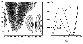Figure 3
In order to determine the duration of the elementary events resolved as the aforementioned two features, we calculated the energy densities Ew (a, t) (Figure 3a) and two scale diagrams for the years 1970 and 1990 (Figure 3b). Astafyeva  showed that the position of the spectral maximum of the energy density Ew (a, t) can be interpreted as the average duration of the elementary event making the major contribution to the energy of the process studied. In our case, the Ew (a, t) pattern and the scale diagrams clearly distinguish a scale of about 10 years, suggesting that the duration of the inferred features with their variation maximums in 1969-1970 and 1989-1990 is no shorter than 10 years.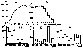Figure 4
As noted above, the variances of distributions on various scales a serve as the measure of fluctuations in the wavelet coefficients. The variation of the variance calculated by formula (9) from the results of the wavelet analysis of SVy is clearly illustrated in Figure 4a. This figure shows that the variance increases until the scale a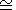40, after which it decreases monotonically. Three features on the scales a = 8-10, a20 and a40 are recognizable in the curve D(a). The variances Dcalculated for these scales with the averaging interval Dt=10 years are shown in Figures 4b, 4c and 4d. The resulting distributions are seen to be scale dependent. No singularities are observed in the distributions of Don larger scales (Figure 4b): the variance increases until the 1940s, after which it decreases monotonically. The histogram is more complicated on the scale a=20 years: several variance extrema are observed, and the Dmaximum at the beginning of the century is followed by a decrease until 1930. Particularly noteworthy is an abrupt decrease in the variance starting from 1950 and lasting until 2000; however, two increase intervals of the variance are observed in 1970-1980 and 1990-2000 against the general decreasing trend of D. The most interesting results were obtained for the small values a=8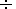10 (Figure 4d).

On these scales, fluctuations in the wavelet coefficients and therefore in the variance are highest, with maximum values of Dbeing observed in the 1970s and 1990s. These years are of particular significance from the standpoint of development of singularities. The distribution of the variance of the initial SV field D, shown in Figure 4e, does not exhibit any anomalous behavior in the years mentioned above, thereby indicating a dynamic origin of these phenomena. Thus, the temporal behavior of the variance of the distribution of wavelet coefficients shows that the scales 8-10 years are most sensitive to the development of a singularity in the SVy field. It is possible that precisely these scales disturb the linear behavior of the sum Zq as a function of the scale a.Figure 5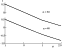Figure 6
Using the results of the wavelet analysis of SVy as an example, we examine the behavior of the sum Zq obtained by formula (11). The values q = -1, 0, 1 and 2 were used in numerical calculations. Examples of the dependence of log Zq on the scale a are given in Figure 5.

The plots presented in Figure 5 indicate that the a dependence of log Zq is nonlinear, i.e., on the whole, the observed process SVy does not possess the property of self-similarity (fractality). However, this dependence is linear for all values of q at large scales ( a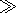2830 ). Therefore, one can state that the process SVy is fractal at large scales a. If this condition is met, the dependence of the function on the rank of moment q (12) determines the mono- or multifractality of the signal analyzed. We constructed the dependences t(q) for large scales a, and their examples for a=30 and 40 are given in Figures 6a and 6b. Noteworthy are two features of these plots: first, the function t(q) is not strictly linear, which is evidence of multifractality of the process, and second, the functions t(q) constructed for different scale values virtually coincide. For this reason, the function t(q) can be considered as a scale-independent measure of the fractal process.

Returning to the results shown in Figure 5, we should note that all plots of the a dependence of log Zq reveal three different structures on the scales a1110, a21030 and a3>30. These structures appear to be due to different sources. The smaller scales can be naturally related to an external source, singularities of the jerk type from internal sources, and noise. As regards the larger scales, they are evidently related to internal sources.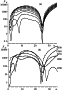Figure 7
As noted above, the energy density of the process Ew (a, b)=W2 (a, b) determines the energy levels of the study process in the space (a, b). Figure 7 presents the energy distribution patterns of the field SVy in various time intervals. Ten scale diagrams are shown in Figure 7a for the period from 1965 to 1975, and Figure 7b presents scale diagrams obtained with step of 10 years for the period from 1960 through 2000. Similar scale diagrams were obtained for the entire study period of SVy. The resulting diagrams allow one to trace the evolution of the energy distribution over scales: the energy density distribution is obviously nonuniform in scale in various observation intervals, and a sharp drop on scales of 20 to 30 years is observed in nearly the entire time interval of SVy.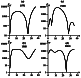Figure 8
Of particular interest are observation periods of the field SVy during which pronounced singularities are observed. Figure 8 compares the scale diagrams for periods of 1970 and 1980 and for periods of 1990 and 2000. The diagrams are seen to strongly differ in their structure and estimated values.

Thus, the results of wavelet analysis show that the SV temporal series are described by a process possessing a wide range of time scales. Apparently, this process is a superposition of several components: a stochastic low-frequency component, several regular variations with possible superimposed singularities, and irregular variations of the noise type.Figure 9
The phase portrayal or attractor of the secular variation is another evidence for its complex structure. Characteristic attractor portrayals obtained by formula (13) are shown in Figure 9. Figure 9f exemplifies a model attractor for a time series representing the sum of two sinusoids with the periods T1 = 60 years and T2 = 20 years. Figures 9a, 9b and 9c present the l=1 SVy phase portrayals for different lengths of temporal series. The SVx and SVz phase portrayals are shown in Figures 9d and 9e. Similar patterns are obtained for greater values of l. Note that the vertical component yields a more complex, intricate portrayal. In this connection, it is important to know the dimension of the attractor providing insights into the dynamic behavior of the process studied. In our case, the attractor has a finite dimension, because its structure does not change with increasing dimension of the surrounding phase space (attractors at various values of m ). The attractor dimension is evidently grater than unity because the phase curve is not closed (the attractor of the dimension 1 has a closed cycle). Apparently, the attractor dimension is no more than 3, because the system becomes unstable (chaotic) at a dimension as large as 4. Usually, trajectories of an attractor of an integer dimension vary in a regular manner, which is not observed in our case. The determination of an exact dimension of an attractor is not a simple problem. In our case, we can only indicate the dimension limits for the phase representation of the dynamic SV system: 2

### 4. Possible Sources of Jerks

Presently, no theory has been developed for elucidating the origin of singularities on the Earth's surface. However, some hypotheses have long been proposed. Thus, in the opinion of Courtillot and Le Mouel , a sharp variation is due to an abrupt change in the generation mode and is associated with additional movements in the upper part of the liquid core that arise in relation to a change in the rotation velocity of the inner part of the core. Possibly existing vertical movements in the liquid core known as upwellings [Voorhies, 1986] can either accelerate or decelerate the Earth's rotation, which should naturally have an effect on the SV generation. Runcorn  believes that singularities in the geomagnetic field can arise due to instabilities in the toroidal field, whereas Hide  regards such variations as a result of the interaction between Alfven waves in the liquid core.

Golovkov et al.  obtained interesting numerical results for the interpretation of jerks in terms of the field of material velocities on the core surface. They showed that the leading mechanism of motions in the liquid core is provided by the rise and descent of material that are described the toroidal component of the velocity field. Vortex motions associated with the toroidal component should be regarded as a secondary effect of the vertical movements.

### 5. Induction Effects

Let the source of a sharp change in the SV field be the following model of a local disturbance: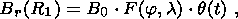(14)

where q is a step function. In this case, the surface field variation should yield a peak the length and shape of which are determined by the conductive mantle.

We assume that F (j, l) describes a compensated source ( S Br dS=0 ) of a small spatial size. After a signal of the chosen geometry having a steplike time dependence passes through the conductive mantle, the field B (R0, t) on the Earth's surface assumes the form consistent with the observed field variation pattern [Braginsky and Fishman, 1977]. Such a theoretical dependence is sharply asymmetric and has a maximum. Near the epicenter, the maximum is attained over the time0.3t. As seen, the role of the conductive mantle does not reduce to the delay of the signal by a certain time (retarding time) and to the Gaussian smoothing of a certain width (smoothing time). The temporal dependence is strongly non-Gaussian.

Characteristic slopes of the temporal dependence before and after the maximum are different. Note that the observation data possess the same properties. It is natural to estimate the mantle conductivity from the characteristic increase time because the post-maximum drop is masked by other smooth variations of the field. The characteristic increase time estimated from observation data is t5 years. Hence, the screening parameter is estimated at t17 years, which differs little from previous estimates of the screening and, accordingly, conductivity [Braginsky and Fishman, 1977] obtained from the analysis of local sources of 60- and 30-year variations amounting to ~10 3 S/m at the core-mantle boundary [Papitashvili et al., 1982].

### 6. Conclusion

The main results of our study reduce to the following.

1. The wavelet analysis applied to SV temporal series of the last 100 years revealed singularities ~10 years long that were most intense in 1970 and 1990.

2. The field SVy was subjected to the dispersion analysis based on the results of wavelet transformations. The analysis detected scales ( a = 810) that are most sensitive to singularities in the SVy temporal series and resolve best the singularities of 1970 and 1990.

3. The results of the SVy wavelet transformation were used for calculating higher moments Zq; their estimates showed that the process analyzed does not possess the property of self-similarity (fractality). The process can be regarded as fractal only on large scales a.

4. The scale diagrams and the higher moments Zq obtained from the SVy wavelet analysis indicate the presence of three different structures depending on scale and most likely due to different sources.

5. The wavelet analysis results allowed us not only to identify singularities in the SVy field but also to trace variations in its energy parameters throughout the time interval studied.

6. The phase portrayal (attractor) constructed for SV confirmed the complexity of the SV structure. The range of the dimension of the SV dynamic system is estimated at 2 7. The origin of the jerks was elucidated from physical considerations on their sources (torsional vibrations and local sources).

8. Based on analysis of the jerks, the characteristic time of screening of the mantle was estimated at t17 years, which yields sH.M.l.m. 10 3 S/m.

#### Acknowledgments

This work was supported by the INTAS Foundation, Grant No. 01-0142, and the Russian Basic Research Foundation.

### References

Alexandrescu, M., D. Gibert, G. Hulot, J.-L. Le Mouel, and G. Saracco, Detection of geomagnetic jerks using wavelet analysis, J. Geophys. Res., 100, 12,557-12,572, 1995.

Alexandrescu, M., D. Gibert, G. Hulot, J.-L. Le Mouel, and G. Saracco, Worldwide wavelet analysis of geomagnetic jerks, J. Geophys. Res., 101, 21,975-21,994, 1996.

Alperovich, L., and V. Zheludev, Wavelet transform as a tool for detection of geomagnetic precursors of earthquakes, Phys. Chem. Earth, 23, 965-967, 1998.

Astafyeva, N. M., The wavelet analysis: Theoretical fundamentals and application examples (in Russian), Usp. Fiz. Nauk, 166, 1145-1170, 1996.

Braginsky, S. I., and V. M. Fishman, The 60-year geomagnetic field variations and the electrical conductivity of the mantle (in Russian), Geomagn. Aeron., 17, 921-926, 1977.

Cay, S. M., and S. L. Marple, Modern Methods of spectral analysis: A review (in Russian), TIIER, 69, (11), 3-51, 1981.

Chui, K., Introduction to Wavelets (in Russian), Mir, Moscow, 2001.

Courtillot, V. E., and J.-L. Le-Mouel, Time variations of the Earth's magnetic field: From daily to secular, Annu. Rev. Earth Planet. Sci., 16, 389-476, 1988.

Daubechies, I., Ten Lectures on Wavelets (in Russian), RKhD, Moscow, 2001.

Daubechies, I., Ten lectures on wavelets, in CBMS Ser. Appl. Math. Soc. Ind. Appl. Math. Pa., 61, Philadelphia, 1992.

De Michelis, P., L. Cafarella, and A. Meloni, A global analysis of the 1991 geomagnetic jerk, Geophys. J. Int., 143, 545-556, 2000.

Dremin, I. M., O. V. Ivanov, and V. A. Nechitailo, Wavelets and their applications (in Russian), Usp. Fiz. Nauk, 171, (5), 465-501, 2001.

Ducruix, J., V. Courtillot, and J.-L. Le Mouel, The late 1960s secular impulse, the eleven year magnetic variation and the electrical conductivity of the deep mantle, Geophys. J. R. Astron. Soc., 61, 73-94, 1980.

Dyakonov, V. P., Wavelets: Theory and Applications (in Russian), Solon-R., Moscow, 2002.

Gilbert, D., M. Holschneider, and J.-L. Le Mouel, Wavelet analysis of the Chandler wobble, J. Geophys. Res., 103, 27,069-27,089, 1998.

Golovkov, V. P., A. O. Simonyan, and S. V. Yakovleva, Calculation of the velocity field at the Earth's core surface from data on geomagnetic jerks (in Russian), Geomagn. Aeron., 36, 114-126, 1996.

Hide, R., A note on short term core-mantle coupling, geomagnetic secular variations impulses, and potential magnetic field invariants as Lagragian trancers of core motions, Phys. Earth Planet. Int., 39 (4), 297-300, 1985.

Ippolitov, I. I., M. V. Kabanov, and S. V. Loginov, Application of the wavelet transformation to the analysis of yearly variations in the surface air temperature in Omsk and solar activity (in Russian), Optika Atm. Okeana, 14, (4), 280-285, 2001.

Ivanov, V. V., and N. M. Rotanova, Wavelet analysis of a magnetic anomaly profile obtained from MAGSAT data (in Russian), Geomagn. Aeron., 40, (2), 78-83, 2000.

Malin, S. R. C., B. M. Hodder, and D. R. Barrachlough, Geomagnetic secular variations: A jerk in 1970, in Publicado on volumen conmemorative 75 aniversario del observatorio del Ebro, pp. 239-256, 1983.

Mallat, S., A theory for multiresolution signal decomposition: the wavelet representation, IEEE Pattern Anal. Machine Intell., 11, 674-693, 1989.

McLeod, M. C., On the geomagnetic jerks of 1969, J. Geophys. Res., 90, 4597-4610, 1985.

Meyer, Y., Wavelets and Operators, Cambridge Univ. Press, 1992.

Papitashvili, N. E., N. M. Rotanova, and V. M. Fishman, Estimating the lower mantle conductivity from 60- and 30-year variations in the geomagnetic field (in Russian), Geomagn. Aeron., 22, 1010-1015, 1982.

Rotanova, N. M., T. N. Bondar, and V. V. Ivanov, Temporal variations in geomagnetic secular variations (in Russian), Geomagn. Aeron., 42, 708-720, 2002.

Runcorn, S. K., Geodynamic implications of short time scale changes in the geomagnetic dynamo, Phys. Earth Planet. Int., 41, 73-77, 1985.

Takens, F., Lecture Notes in Mathematics, D. Rand and I.-S. Young, Eds., Springer-Verlag, New York, 1981.

Voorhies, C. V., Steady flows at the top of Earth's core derived from geomagnetic field models, J. Geophys. Res., 91, 1244-1266, 1986.

Load files for printing and local use. This document was generated by TeXWeb (Win32, v.1.3) on December 27, 2003.